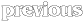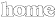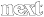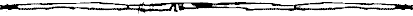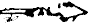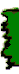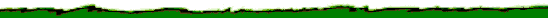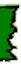THINGS TO SEEK FOR . . .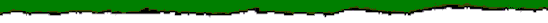Notes on the coordinate system: The Coordinates are in the order ( y, x ) where y is between 1 and 61 and x is between 0 and 63. This is how I generated them for some odd reason and I'm not going to change it now!
 Guidance
 ( 1,30) Deadhenge ( 1,62) The Tower Of Lorgrim ( 6,16) A Lith In The Domain Of The Lost ( 8,61) The Tower Of Fadrath ( 9,50) Droonhenge (10, 6) A Lith In The Domain Of Moon (10,12) The Tower Of Lothoril (10,36) The Tower Of Ugrorn (10,41) The Lith Of Grarg (11,51) A Lith In The Domain Of Ithril (12,45) The Tower Of Dreams (15, 6) A Lith In The Domain Of Mirrow (16,55) The Lith Of Whispers (19,52) A Lith In The Domain Of Ithril (22,36) A Lith In The Domain Of Kor (22,44) Korhenge (23,54) Coomhenge (23,58) The Tower Of Whispers (25,12) The Tower Of Coroth
 (27,16) The Lith Of Coroth (28, 7) The Tower Of Ashimar (28,37) The Tower Of Kor (29,44) The Tower Of Marakith (31,60) The Tower Of Kumar (31,61) The Tower Of Athoril (32,18) The Tower Of Dodrak (34,49) The Lith Of Athoril (34,57) A Lith In The Domain Of The Targ (41,12) The Tower Of The Moon (46,60) The Lith Of Dregrim (48,61) The Tower Of Coom (49,14) A Lith In The Domain Of Gard (50,38) The Tower Of Morning (53,53) The Tower Of Trorn (55, 6) The Tower Of Elenil (55,14) A Lith In The Domain Of Gard (61,36) The Tower Of Corelay

 (11, 1) Moonhenge (11,14) A Ruin In The Domain Of Lothoril (25,62) A Ruin In The Domain Of Kumar (31,25) A Ruin In The Domain Of Valethor (42,52) A Ruin In The Domain Of Athoril (43,26) The Ruin Of Mitharg (46,39) A Ruin In The Domain Of Dawn (48,44) A Ruin In The Domain Of Corelay (52,61) Trornhenge

 Waters of Life
 ( 2,46) Lake Lor ( 2,52) Lorhenge ( 2,61) Lake Lorgrim ( 4,35) Lake Rathrorn ( 5, 3) Lake Gloom ( 8,62) Lake Fadrath ( 9,21) Lake Ugrak ( 9,46) A Lake In The Domain Of Ithril ( 9,48) A Lake In The Domain Of Ithril (10, 3) A Lith In The Domain Of Moon (10,59) A Lake In The Domain Of Droon (10,62) A Lake In The Domain Of Lorgrim (11,12) Lake Lothoril (11,34) Lake Ugrorn (11,58) Lake Droon (11,61) A Lake In The Domain Of Ithril (14,43) Lake Ithril (14,56) A Lake In The Domain Of Ithril (16,12) A Lith In The Domain Of Glorim (17,21) A Lith In The Domain Of Kor (17,25) Lake Moon (18, 9) Lake Mirrow (18,56) Lake Ithrorn (19,50) A Lake In The Domain Of Ithril (20,16) Lake Korkith (20,45) A Lake In The Domain Of Ithril (21,25) Lake Kor (21,33) A Lith In The Domain Of Kor (21,45) A Lake In The Domain Of Ithril (24,19) Lake Gorgrath (24,52) Lake Herath (25, 7) Lake Ogrim (25,13) Lake Dodrak (26, 4) Lake Toomog (26,40) A Lake In The Domain Of Kor (28,46) Lake Kumar (29,26) Lake Valethor (31,15) A Lake In The Domain Of Valethor (32,40) Lake Marakith
 (32,63) A Lake In The Domain Of The Targ (33,62) A Lake In The Domain Of The Targ (35,27) Bloodhenge (35,35) A Lith In The Domain Of Marakith (36,17) The Lith Of Blood (36,41) A Lake In The Domain Of Marakith (36,54) Lake Utarg (38,26) Lake Blood (39,34) Lake Thrall (39,51) Lake Athoril (40, 2) Lake Ashimar (40,58) A Lith In The Domain Of Athoril (44,22) Lake Mitharg (44,40) Lake Dawn (46,30) Lake Shimeril (46,46) A Lake In The Domain Of Dawn (47,60) Lake Dregrim (48,38) A Lake In The Domain Of Rorath (48,48) A Lake In The Domain Of Trorn (49, 4) Lake Torkren (49,36) A Lake In The Domain Of Rorath (49,51) Lake Trorn (51,12) Lake Gard (51,47) A Lake In The Domain Of Corelay (53, 9) Lake Silence (53,35) A Lake In The Domain Of Morning (53,38) A Lake In The Domain Of Morning (54, 7) Lake Elenil (55,49) A Lake In The Domain Of Corelay (57,26) Lake Odrark (57,51) A Lake In The Domain Of Corelay (58, 2) A Lake In The Domain Of Torkren (58, 6) A Lake In The Domain Of Silence (58,54) A Lake In The Domain Of Corelay (59,47) A Lake In The Domain Of Corelay (60,23) Lake Rorath (60,56) A Lake In The Domain Of Corelay (61, 1) A Lake In The Domain Of Torkren (61,38) A Lake In The Domain Of Corelay

 Hand of Dark
 ( 2,13) A Snowhall In The Domain Of The Lost ( 2,34) A Snowhall In The Domain Of Rathrorn ( 2,63) A Snowhall In The Domain Of Lorgrim (10,52) A Snowhall In The Domain Of Ithril (11, 7) The Cavern Of Moon (11,55) The Cavern Of Ithril (15, 9) The Ruin Of Glorim (24, 2) Mirrowhenge (26,61) The Cavern Of Kumar (30, 1) A Snowhall In The Domain Of Ogrim (32,58) A Ruin In The Domain Of The Targ
 (34,52) A Cavern In The Domain Of The Targ (35,15) The Ruin Of Dodrak (39,61) A Ruin In The Domain Of The Targ (43, 5) The Cavern Of Torkren (44,61) The Cavern Of Dregrim (47, 2) A Snowhall In The Domain Of Torkren (47,12) A Ruin In The Domain Of Gard (55,62) The Cavern Of Trorn (58,34) The Cavern Of Corelay (60,35) A Henge In The Domain Of Corelay (61,19) The Ruin Of Brith

 Cup of Dreams
 ( 1,40) The Lith Of Rathrorn ( 3,20) A Lith In The Domain Of The Lost ( 4,18) Weirdhenge ( 5,57) The Snowhall Of Lorgrim ( 8, 1) A Snowhall In The Domain Of Moon ( 9, 4) A Snowhall In The Domain Of Lothoril ( 9,11) A Henge In The Domain Of Lothoril ( 9,27) A Lith In The Domain Of Despair (10, 4) A Lith In The Domain Of Moon (12, 4) A Lith In The Domain Of Moon (12, 5) A Lith In The Domain Of Moon (19,59) The Lith Of Ithrorn (20,32) A Lith In The Domain Of Kor (21,35) A Lith In The Domain Of Kor (23,35) A Lith In The Domain Of Kor (24,36) A Lith In The Domain Of Kor
 (26,53) The Lith Of Herath (31,16) The Lith Of Valethor (35,41) The Lith Of Marakith (35,47) Thrallhenge (37,50) A Lith In The Domain Of Athoril (42,40) Dawnhenge (44,33) A Lith In The Domain Of Iserath (47,14) The Lith Of Mitharg (47,52) A Lith In The Domain Of Trorn (48,16) Gardhenge (51,14) A Lith In The Domain Of Gard (53,14) The Lith Of Gard (54,59) A Lith In The Domain Of Trorn (57,57) A Henge In The Domain Of Corelay (59, 1) A Lith In The Domain Of Torkren (60,60) A Lith In The Domain Of Corelay

 The Ice Crown
 (5, 26) Tower of Doom